# HOW TO SQUARE & CUBE A NUMBER-LEARN SERIES:

Hi Bankersdaily Aspirants,

Learning is a step by step process and you should gradually progress in that to ace the process. As you all know we have started a new Learn Series to help with your preparations for various exams like IBPS PO , IBPS RRB Scale I officer , IBPS RRB Office Assistant , IBPS Clerk , IBPS SO , SBI PO , SBI Clerk , SBI SO and many other exams in this type. Nevertheless this series will also contribute you all the tips and tricks to every miscellaneous sections and also all the important topics which are asked in the exams. If you haven’t looked at the Learn series , Please Click the below link to learn the new concepts.

### INTRODUCTION:

In Competitive exams like IBPS which is becoming tougher year by year,Simplification is the only topic in which we can score easily and obtain 5 marks less than 5 minutes.Today we are going to look on how to square & cube a number anyway this is not going to ask as a separate question but it may be in Simplification and other miscellaneous topics also

### HOW TO SQUARE A NUMBER :

To square a number there is only two steps

1)78²

Whenever a number is given,split the number into two parts unit digit as 1 part and remaining number as tens part

78 =   7 / 8

Step 1: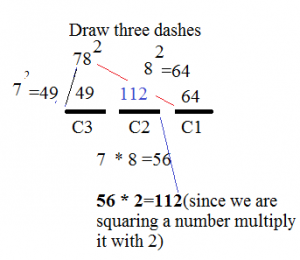Step 2:

Keep the Unit digit in each Column & Carry the remaining number to the next Column.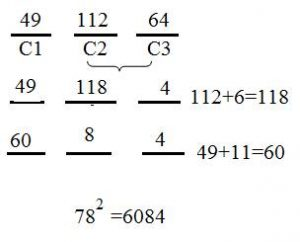2)128²

Whenever a number is given,split the number into two parts unit digit as 1 part and remaining number as tens part

128=12 /  8

STEP 1: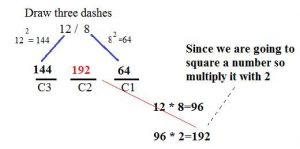STEP 2:

Keep the Unit digit in each Column & Carry the remaining number to the next Column.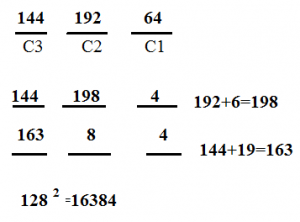### HOW TO CUBE A NUMBER:

To Cube a Number there is one more Step additional to the above 2 steps which we had seen while squaring a number.

1)78³

When ever a number is given just divide the number into 2 parts unit digit as 1 part and remaining number as 2nd part.

7 / 8

STEP 1: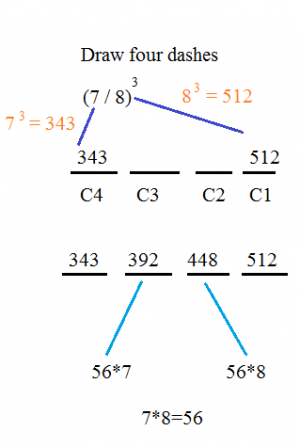STEP 2:

Double the digits in C2 and C3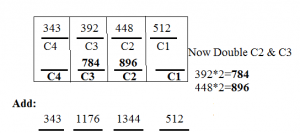STEP 3:

Keep the Unit digit in each column and carry over the remaining number to the next column: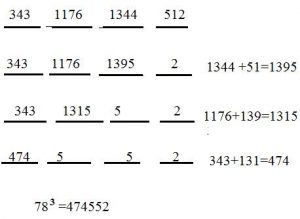2)128³

When ever a number is given just divide the number into 2 parts unit digit as 1 part and remaining number as 2nd part.

(12 / 8)³

STEP 1: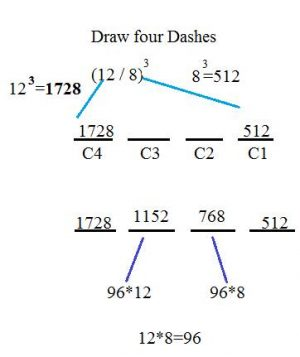STEP 2:

Double the digits in C2 and C3STEP 3:

Keep the Unit digit in each column and carry over the remaining number to the next column: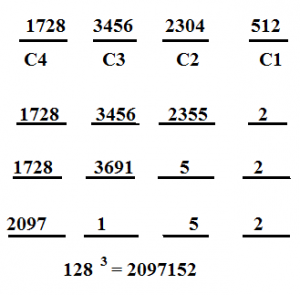## Related Articles:

### TIPS AND TRICKS TO CRACK REASONING SECTION IN IBPS PO PRELIMS 2017

PIPES AND CISTERN PART 2-LEARN SERIES